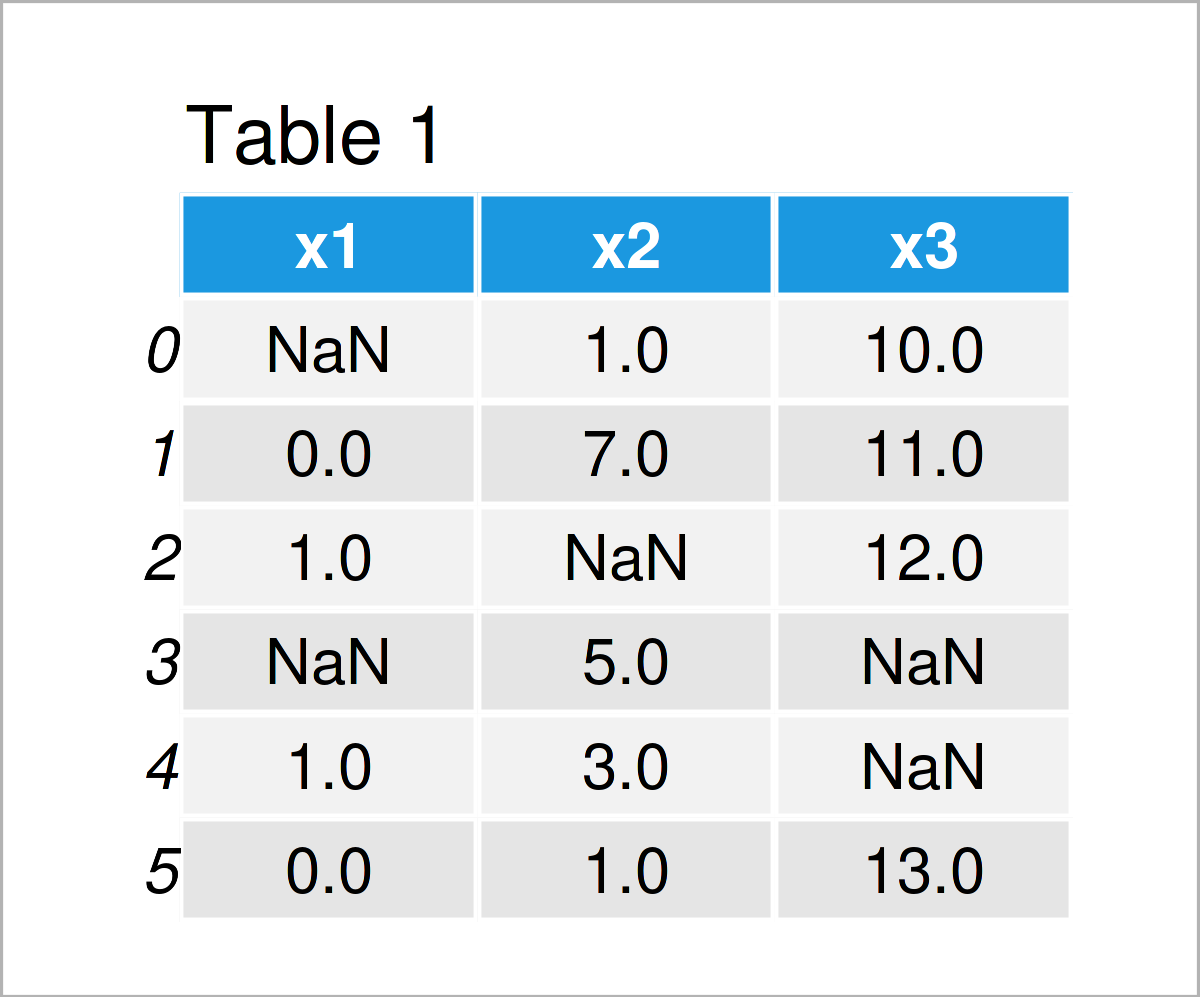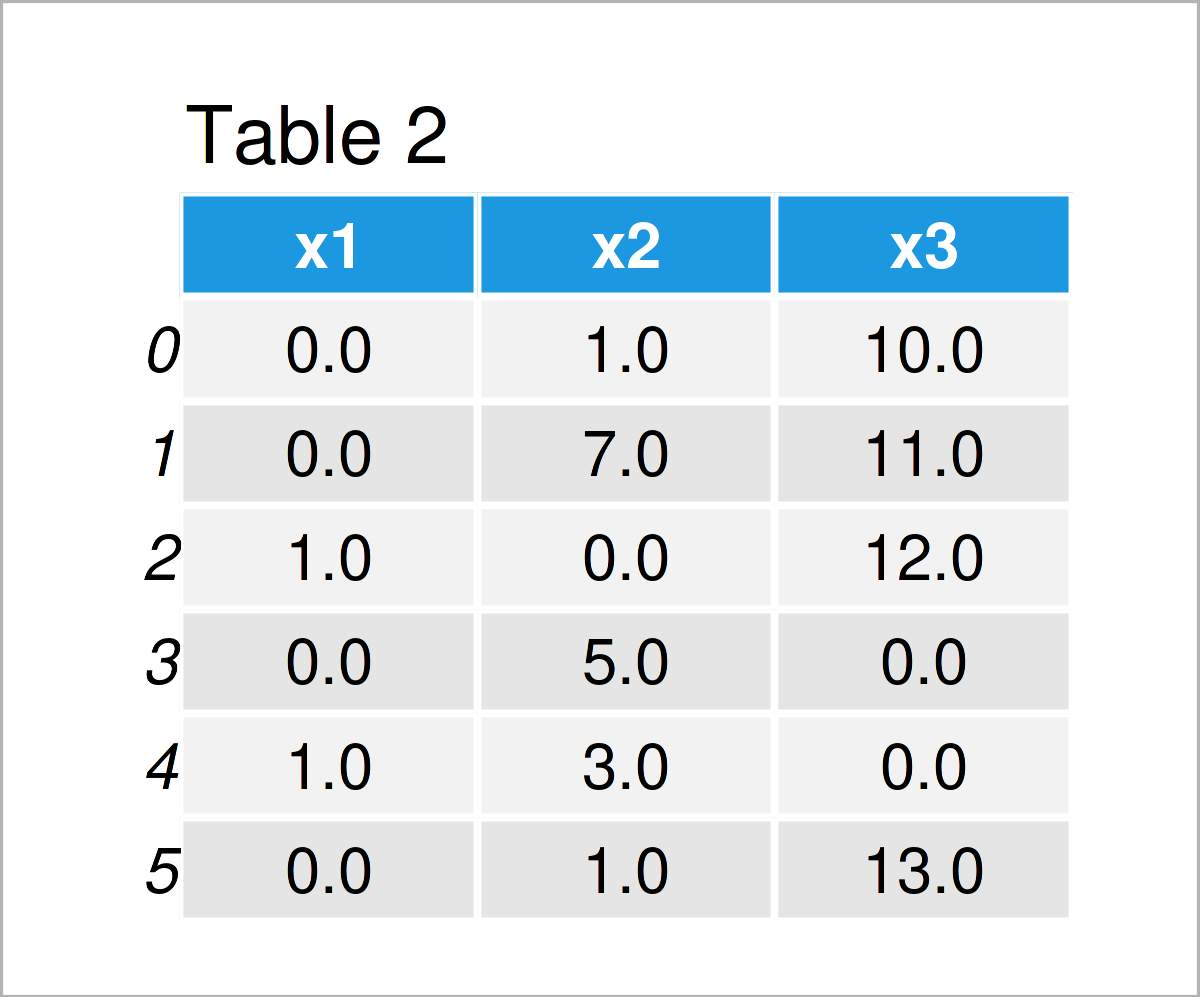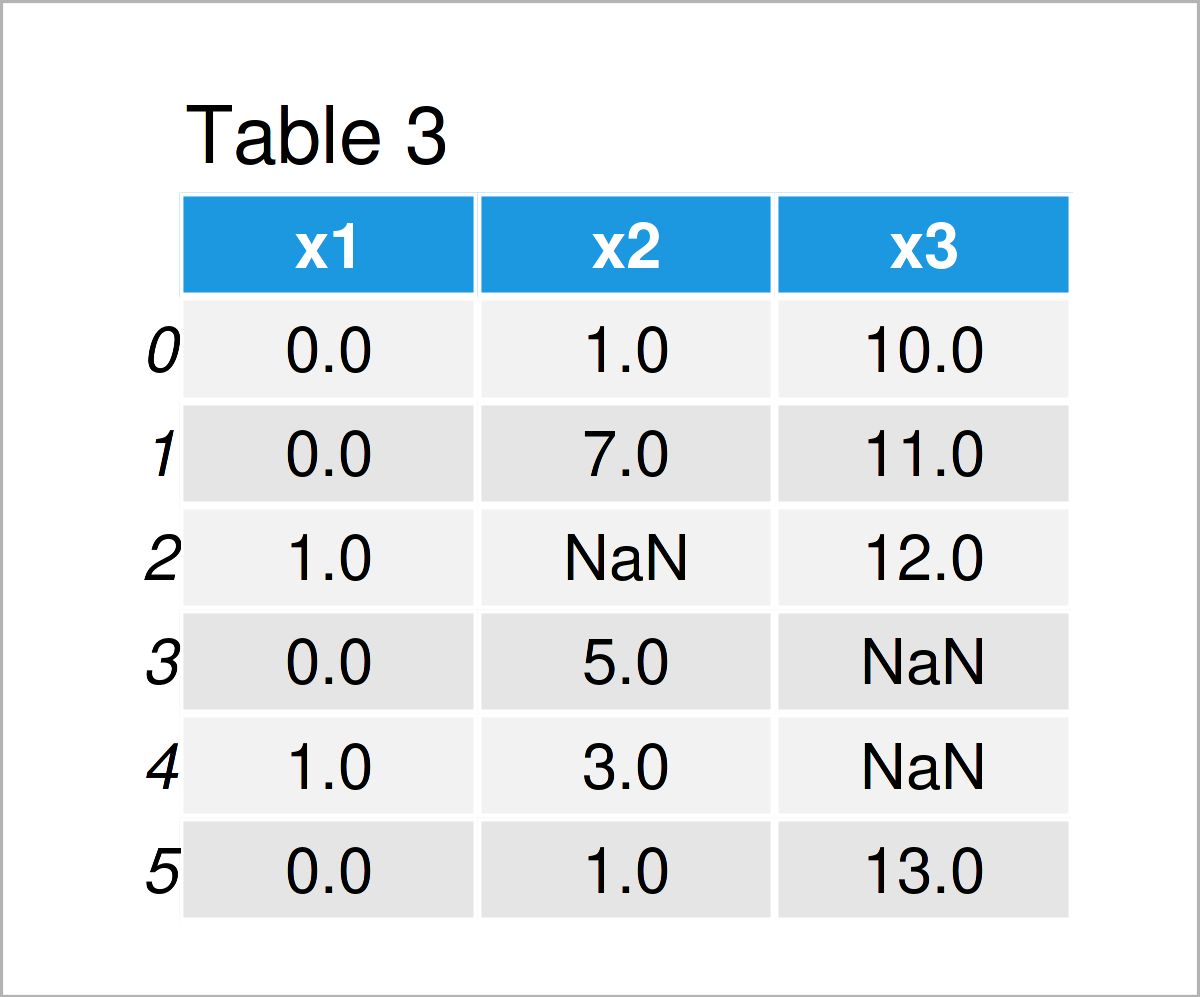# Replace NaN with 0 in pandas DataFrame in Python (2 Examples)

In this tutorial you’ll learn how to exchange NaN values in a pandas DataFrame by 0 in the Python programming language.

The page looks as follows:

Let’s dive into it:

## Exemplifying Data & Software Libraries

We first need to load the pandas library, to be able to use the functions that are included in the library:

`import pandas as pd                                         # Import pandas`

The following data will be used as basement for this Python programming tutorial:

```data = pd.DataFrame({'x1':[float('NaN'), 0, 1, float('NaN'), 1, 0],  # Create DataFrame
'x2':[1, 7, float('NaN'), 5, 3, 1],
'x3':[10, 11, 12, float('NaN'), float('NaN'), 13]})
print(data)                                                # Print example DataFrame```Have a look at the previous table. It shows that our example data is constructed of six rows and three columns. All of these columns contain NaN values.

## Example 1: Convert NaN to Zero in Entire pandas DataFrame

In Example 1, I’ll explain how to replace NaN values in all columns of a pandas DataFrame in Python.

For this task, we can apply the fillna function as shown below:

```data_new1 = data.fillna(0)                                 # Substitute NaN in all columns
print(data_new1)                                           # Print DataFrame with zeros```By executing the previous code we have created Table 2, i.e. a new pandas DataFrame called data_new1 that contains zeros instead of NaN values.

## Example 2: Convert NaN to Zero in Specific Column of pandas DataFrame

In Example 1, we have exchanged all NaN values in each column of our pandas DataFrame.

The following Python syntax demonstrates how to convert only the NaN values of one specific variable to 0.

Have a look at the Python syntax below:

```data_new2 = data.copy()                                    # Create copy of input DataFrame
data_new2['x1'] = data_new2['x1'].fillna(0)                # Substitute NaN in single column
print(data_new2)                                           # Print DataFrame with zeros in single column```After running the previous Python programming syntax the DataFrame shown in Table 3 has been created.

As you can see, we have only replaced the NaNs in the column x1.

## Video & Further Resources on this Topic

I have recently released a video on my YouTube channel, where I explain the Python syntax of this article in some more detail. You can find the video below:

Please accept YouTube cookies to play this video. By accepting you will be accessing content from YouTube, a service provided by an external third party.If you accept this notice, your choice will be saved and the page will refresh.

Would you like to learn more about the handling and the substitution of NaN values in a pandas DataFrame by the value 0? Then I recommend watching the following video on the YouTube channel of Minsuk Heo. He shows similar examples as illustrated by the Python programming codes of this tutorial in the video.

Please accept YouTube cookies to play this video. By accepting you will be accessing content from YouTube, a service provided by an external third party.If you accept this notice, your choice will be saved and the page will refresh.

In addition to the video, you might want to have a look at the other tutorials on this website. Some articles about the substitution of NaN values in a pandas DataFrame by the value 0 are shown below:

In summary: At this point you should have learned how to substitute NaN values in a pandas DataFrame by the value 0 in the Python programming language. If you have further questions, please tell me about it in the comments.

Subscribe to the Statistics Globe Newsletter